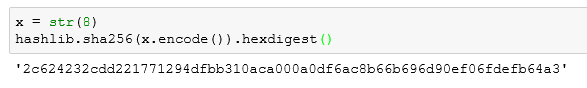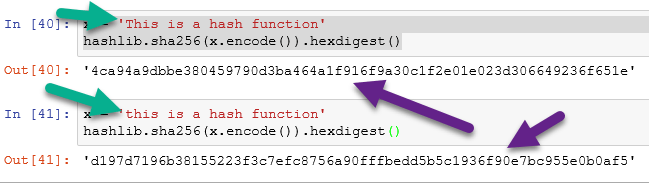# Python: Create a Blockchain Hash Function

If you are at all like me, reading about a concept is one thing. Actually practicing it though, that helps me to actually understand it. If you have been reading my blockchain tutorial, or if you came from an outside tutorial, then you have undoubtedly read enough about cryptographic hashes.

( if you are unfamiliar with crytographic hashes, you can reference my tutorial on them here: Blockchain: Cryptographic Hash )

For this example, I am using the Anaconda Python 3 distribution.

Like most things in Python, creating a hash is as simple as importing a library someone has already created for us. In this case, that library is: hashlib

So our first step is to import hashlib

`import hashlib`

Now let us take a moment to learn the syntax require to create a cryptographic hash with hashlib. In this example, I am using the SHA 256 hashing algorithm. I am using this because it is the same algorithm used by BitCoin.

Here is the syntax used

`hashlib.sha256(string.encode()).hexdigest()`

To understand the syntax, we are calling the hashlib method sha256(): hashlib.sha256()

Inside the brackets, we are entering the string we want to encode in the hash. Yes it must be a string for this function to work.

Still inside the brackets we use the method .encode() to (surprise, surprise) ENCODE the string as a hash

Finally, I added the method .hexdigest() to have the algorithm return our hash in hexadecimal format. This format will help in understanding future lessons on blockchain mining.

So in the example below, you can see that I assigned the variable x the string ‘doggy’. I then passed x to our hash function. The output can be seen below.Now a hash can hold much more than just a simple word. Below, I have passed the Gettysburg Address to the hashing function.

(**note the ”’ ”’ triple quotes. Those are used in Python if your string takes up more than one line **)Now I try passing a number. You will notice I get an error.To avoid the error, I turn the integer 8 into a string with the str() functionBelow I concatenation a string and an integer.Last I want to show the avalanche effect of the hash function.By simply changing the first letter from an uppercase T to a lowercase t the hash changes completely. This is a requirement for hashing functions. If the hash did not change dramatically from a small change to the string, it would be easy to reverse engineer the hash. This is known as the avalanche effect.